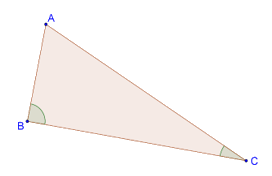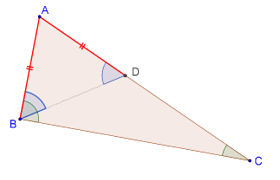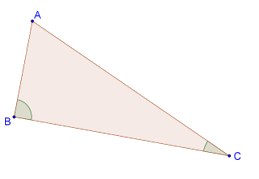# Relative Magnitudes of Sides and Angles

Relative Magnitudes of Sides and Angles

We have seen that in a triangle, the angles opposite two equal sides are equal. Conversely, if two angles are equal in a triangle, then the sides opposite these angles are equal.

What if in a triangle, two sides (or angles) are unequal? Can we then talk about the relative magnitudes of the angles (or sides) opposite these?

Theorem: If two sides of a triangle are unequal, then the greater side has the greater angle opposite to it.

Take a look at the following figure of a triangle ABC, in which AC is larger than AB. We have to show that ∠B is greater than ∠C.Proof: Since AC is larger than AB, we can take a point D on AC such that AB = AD, as shown below:In ∆ABD, since AB = AD, the angles opposite these equal sides must be equal: ∠ABD = ∠ADB. Now, note that ∠ADB is an exterior angle for ∆BDC, which means that ∠ADB > ∠C. Thus, ∠ABD > ∠C.

Finally, since ∠B must be greater than ∠ABD, we have: ∠B > ∠C.

The converse of this theorem also holds.

Theorem: If two angles of a triangle are unequal, then the greater angle has the greater side opposite to it. Consider the following triangle, for which it is given that ∠B > ∠C. We have to show that AC > AB.Proof: Note that AC cannot be equal to AB, since then ∠B would have been equal to ∠C. Similarly, AC cannot be less than AB, since then ∠B would have been less than ∠C (we have just proven this result). Thus, the only possibility is that AC is greater than AB.

grade 9 | Questions Set 1
grade 9 | Questions Set 2
More Important Topics
Numbers
Algebra
Geometry
Measurement
Money
Data
Trigonometry
Calculus
More Important Topics
Numbers
Algebra
Geometry
Measurement
Money
Data
Trigonometry
Calculus